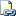# Expressions (Transact-SQL)

Is a combination of symbols and operators that the SQL Server Database Engine evaluates to obtain a single data value. Simple expressions can be a single constant, variable, column, or scalar function. Operators can be used to join two or more simple expressions into a complex expression.Transact-SQL Syntax Conventions

## Syntax

``````{ constant | scalar_function | [ table_name. ] column | variable
| ( expression ) | ( scalar_subquery )
| { unary_operator } expression
| expression { binary_operator } expression
| ranking_windowed_function | aggregate_windowed_function
}
``````

## Arguments

Term

Definition

constant

Is a symbol that represents a single, specific data value. For more information, see Constants (Transact-SQL).

scalar_function

Is a unit of Transact-SQL syntax that provides a specific service and returns a single value. scalar_function can be built-in scalar functions, such as the SUM, GETDATE, or CAST functions, or scalar user-defined functions.

[ table_name. ]

Is the name or alias of a table.

column

Is the name of a column. Only the name of the column is allowed in an expression.

variable

Is the name of a variable, or parameter. For more information, see DECLARE @local_variable (Transact-SQL).

( expression )

Is any valid expression as defined in this topic. The parentheses are grouping operators that make sure that all the operators in the expression within the parentheses are evaluated before the resulting expression is combined with another.

( scalar_subquery )

Is a subquery that returns one value. For example:

SELECT MAX(UnitPrice)

FROM Products

{ unary_operator }

Is an operator that has only one numeric operand:

• + indicates a positive number.

• - indicates a negative number.

• ~ indicates the one's complement operator.

Unary operators can be applied only to expressions that evaluate to any one of the data types of the numeric data type category.

{ binary_operator }

Is an operator that defines the way two expressions are combined to yield a single result. binary_operator can be an arithmetic operator, the assignment operator (=), a bitwise operator, a comparison operator, a logical operator, the string concatenation operator (+), or a unary operator. For more information about operators, see Operators (Transact-SQL).

ranking_windowed_function

Is any Transact-SQL ranking function. For more information, see Ranking Functions (Transact-SQL).

aggregate_windowed_function

Is any Transact-SQL aggregate function with the OVER clause. For more information, see OVER Clause (Transact-SQL).

## Expression Results

For a simple expression made up of a single constant, variable, scalar function, or column name: the data type, collation, precision, scale, and value of the expression is the data type, collation, precision, scale, and value of the referenced element.

When two expressions are combined by using comparison or logical operators, the resulting data type is Boolean and the value is one of the following: TRUE, FALSE, or UNKNOWN. For more information about Boolean data types, see Comparison Operators (Transact-SQL).

When two expressions are combined by using arithmetic, bitwise, or string operators, the operator determines the resulting data type.

Complex expressions made up of many symbols and operators evaluate to a single-valued result. The data type, collation, precision, and value of the resulting expression is determined by combining the component expressions, two at a time, until a final result is reached. The sequence in which the expressions are combined is defined by the precedence of the operators in the expression.

## Remarks

Two expressions can be combined by an operator if they both have data types supported by the operator and at least one of these conditions is true:

• The expressions have the same data type.

• The data type with the lower precedence can be implicitly converted to the data type with the higher data type precedence.

If the expressions do not meet these conditions, the CAST or CONVERT functions can be used to explicitly convert the data type with the lower precedence to either the data type with the higher precedence or to an intermediate data type that can be implicitly converted to the data type with the higher precedence.

If there is no supported implicit or explicit conversion, the two expressions cannot be combined.

The collation of any expression that evaluates to a character string is set by following the rules of collation precedence. For more information, see Collation Precedence (Transact-SQL).

In a programming language such as C or Microsoft Visual Basic, an expression always evaluates to a single result. Expressions in a Transact-SQL select list follow a variation on this rule: The expression is evaluated individually for each row in the result set. A single expression may have a different value in each row of the result set, but each row has only one value for the expression. For example, in the following SELECT statement both the reference to ProductID and the term 1+2 in the select list are expressions:

``````USE AdventureWorks2012;
GO
SELECT ProductID, 1+2
FROM Production.Product;
GO
``````

The expression 1+2 evaluates to 3 in each row in the result set. Although the expression ProductID generates a unique value in each result set row, each row only has one value for ProductID.

#### Reference

CASE (Transact-SQL)

CAST and CONVERT (Transact-SQL)

COALESCE (Transact-SQL)

Data Type Precedence (Transact-SQL)

Data Types (Transact-SQL)

Built-in Functions (Transact-SQL)

LIKE (Transact-SQL)

NULLIF (Transact-SQL)

SELECT (Transact-SQL)

WHERE (Transact-SQL)

#### Concepts

Data Type Conversion (Database Engine)